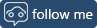# ISBN Functionsby | July 31, 2007 | Classic ASP Web Development

ISBN-10 to ISBN-13 Conversion and Check Digit Calculation

fnisbn13

This Classic ASP function will convert your old 10 digit ISBN numbers to the new ISBN-13 standard. It requires one parameter: either a 9 or 10 digit ISBN-10.

`function fnisbn13(strparam1)	vari=0	varv=0	varn=0	varc=""	varResult=""	vars12=""	varISBN10=strparam1	varlen=len(varISBN10)	if varlen<9 or varlen>10 then		varResult="ERROR"	else		vars12="978"+left(varISBN10,9)		for vari=0 to 11			if varResult="" then				varc=mid(vars12,vari+1,1)				if varc>="0" and varc<="9" then					varv=varc-0					if vari mod 2<>0 then varv=3*varv					varn=varn+varv				end if			else				varResult="ERROR"			end if		next		if varResult="" then			varn=varn mod 10			if varn<>0 then varn=10-varn			varResult=vars12 & varn		end if	end if	fnisbn13=varResultend function`

## Related Posts

### Intermittent "Unable to read data from the transport connection: net_io_connectionclosed" errors

by John Avis | May 6, 2020

If you are having intermittent problems sending email in .NET using System.Net.Mail consider switching libraries.

### 500 Internal Server Error after migrating from IIS 7.5 to IIS 10

by John Avis | November 4, 2019

As support ends for Microsoft Windows Server 2008 I have recently gone through migrating some websites to a new server running Windows Server 2016 and IIS 10 but some of the websites did not work.

### tagInput: A simple jQuery plugin for tag entry using Bootstrap 4

by John Avis | October 15, 2019

For a website project I needed a way to enter multiple tags. I just wanted something simple that I could easily modify to suit my own needs, so I wrote my own.

## Tags